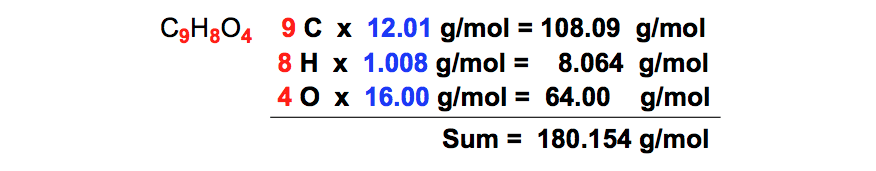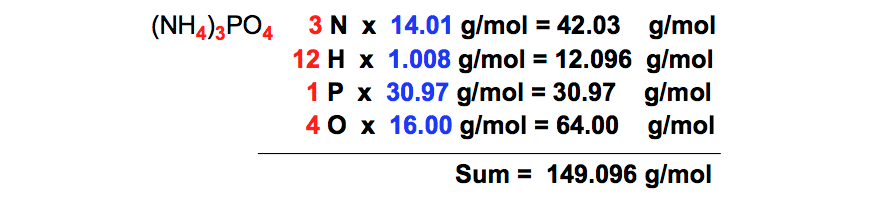Ch.2 - Atoms & ElementsWorksheetSee all chapters
 Ch.1 - Intro to General Chemistry 2hrs & 53mins 0% complete Worksheet Ch.2 - Atoms & Elements 2hrs & 49mins 0% complete Worksheet Ch.3 - Chemical Reactions 3hrs & 25mins 0% complete Worksheet BONUS: Lab Techniques and Procedures 1hr & 38mins 0% complete Worksheet BONUS: Mathematical Operations and Functions 47mins 0% complete Worksheet Ch.4 - Chemical Quantities & Aqueous Reactions 3hrs & 55mins 0% complete Worksheet Ch.5 - Gases 3hrs & 47mins 0% complete Worksheet Ch.6 - Thermochemistry 2hrs & 28mins 0% complete Worksheet Ch.7 - Quantum Mechanics 2hrs & 35mins 0% complete Worksheet Ch.8 - Periodic Properties of the Elements 1hr & 57mins 0% complete Worksheet Ch.9 - Bonding & Molecular Structure 2hrs & 5mins 0% complete Worksheet Ch.10 - Molecular Shapes & Valence Bond Theory 1hr & 31mins 0% complete Worksheet Ch.11 - Liquids, Solids & Intermolecular Forces 3hrs & 40mins 0% complete Worksheet Ch.12 - Solutions 2hrs & 17mins 0% complete Worksheet Ch.13 - Chemical Kinetics 2hrs & 22mins 0% complete Worksheet Ch.14 - Chemical Equilibrium 2hrs & 26mins 0% complete Worksheet Ch.15 - Acid and Base Equilibrium 4hrs & 42mins 0% complete Worksheet Ch.16 - Aqueous Equilibrium 3hrs & 48mins 0% complete Worksheet Ch. 17 - Chemical Thermodynamics 1hr & 44mins 0% complete Worksheet Ch.18 - Electrochemistry 2hrs & 58mins 0% complete Worksheet Ch.19 - Nuclear Chemistry 1hr & 33mins 0% complete Worksheet Ch.20 - Organic Chemistry 3hrs 0% complete Worksheet Ch.22 - Chemistry of the Nonmetals 2hrs & 1min 0% complete Worksheet Ch.23 - Transition Metals and Coordination Compounds 1hr & 54mins 0% complete Worksheet

# Calculating Molar Mass

See all sections
Sections
Periodic Table
Mole Concept
Isotopes
Mass Spectrometry
Subatomic Particles
Atomic Theory
Thomson's Cathode Ray Tube Experiment
Oil Drop Experiment
Gold Foil Experiment
End of Chapter 2 ProblemsJules Bruno

Molar mass or molecular weight is a physical property that represents the mass of a substance divided by the amount of that substance. The common units are typically in grams per mole (g/mol), while the si units are in kilograms per mole (kg/mol).

How to Calculate Molar Mass

In order to determine the molar mass of a compound we must determine the number of atoms for the elements within a given compound.

For example, if we are asked to determine the molar mass of glucose, C6H12O6, we can solve with steps shown below.

STEP 1: Count the number of each element within the compound to get 6 carbons, 12 hydrogens and 6 oxygens.Number of atoms

STEP 2: Find the atomic mass for each chemical element from the periodic table.Periodic Table (Atomic Masses)

STEP 3: Multiply the number of each element by their atomic masses taken from the periodic table.Addition of Atomic Masses

STEP 4: Add up all the masses you got from multiplication together to give the overall molar mass of the compound.Molar Mass of Glucose

From simple compounds such as CO2, H2O, or ammonia to more complex compounds such as proteins, amino acids, acetone or acetic acid we now calculate their molar masses.

PRACTICE 1: Calculate the formula weight of aspirin, C9H8O4, based on the given molecular formula.Molar Mass (Aspirin)

Aspirin contains 9 carbon, 8 hydrogen and 4 oxygen atoms with a total mass of 180.154 g/mol.

PRACTICE 2: Calculate the molar mass of silver nitrate, AgNO3.Molar Mass (Silver Nitrate)

Silver nitrate contains 1 silver, 1 nitrogen and 3 oxygen atoms with a total mass of 169.88 g/mol.

PRACTICE 3: Determine the molecular weight of ammonium phosphate, (NH4)3PO4Molar Mass (Ammonium Phosphate)

Ammonium phosphate contains 3 nitrogens, 12 hydrogens, 1 phophate and 4 oxygen atoms with a total mass of 149.096 g/mol.

Why is the molar mass so important?

Molar mass serves as the foundation for many calculations and ideas found in chemistry. It serves as the bridge that connects together concepts in stoichiometry such as mass conversions, moles, avogadro’s number (6.022 x 1023) or in dimensional analysis such as density, amu, and moles.

In addition we discuss the average molar mass or average molecular mass of compounds when we cover isotopes, the colligative properties such as boiling point elevation or freezing point depression, and the rates of gases through effusion and diffusion.Jules Bruno

Jules felt a void in his life after his English degree from Duke, so he started tutoring in 2007 and got a B.S. in Chemistry from FIU. He’s exceptionally skilled at making concepts dead simple and helping students in covalent bonds of knowledge.